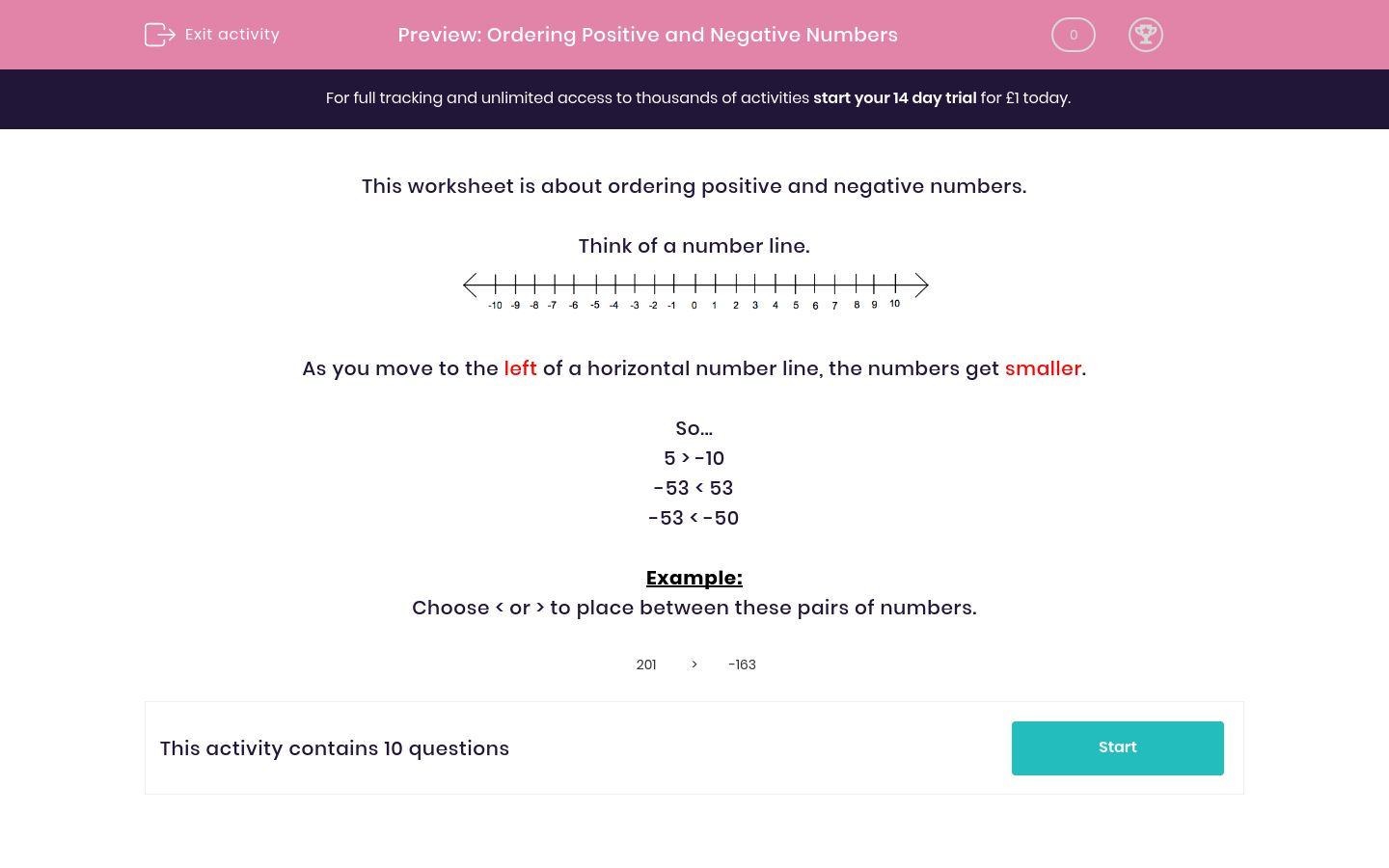# Ordering Positive and Negative Numbers

In this worksheet, students increase their skills in ordering positive and negative numbers.Key stage:  KS 2

Curriculum topic:   Maths and Numerical Reasoning

Curriculum subtopic:   Place Value

Difficulty level:### QUESTION 1 of 10

This worksheet is about ordering positive and negative numbers.

Think of a number line.As you move to the left of a horizontal number line, the numbers get smaller.

So...

5 > -10

-53 < 53

-53 < -50

Example:

Choose < or > to place between these pairs of numbers.

201
 >
-163

Choose < or >  to place between these pairs of numbers.

−375 948
<

>

Choose < or > to place between these pairs of numbers.

380 −299
<

>

Choose < or > to place between these pairs of numbers.

−349 437
<

>

Choose < or > to place between these pairs of numbers.

−14 −56
<

>

Choose < or > to place between these pairs of numbers.

5 204
<

>

Choose < or > to place between these pairs of numbers.

432 −266
<

>

Choose < or > to place between these pairs of numbers.

346 340
<

>

Choose < or > to place between these pairs of numbers.

-81 0
<

>

Choose < or > to place between these pairs of numbers.

−365 −104
<

>

Choose < or > to place between these pairs of numbers.

201 -163
<

>

• Question 1

Choose < or >  to place between these pairs of numbers.

−375 948
<
EDDIE SAYS
The inequality arrow must point at the smaller number
• Question 2

Choose < or > to place between these pairs of numbers.

380 −299
>
EDDIE SAYS
The inequality arrow must point at the smaller number
• Question 3

Choose < or > to place between these pairs of numbers.

−349 437
<
EDDIE SAYS
The inequality arrow must point at the smaller number
• Question 4

Choose < or > to place between these pairs of numbers.

−14 −56
>
EDDIE SAYS
The inequality arrow must point at the smaller number
• Question 5

Choose < or > to place between these pairs of numbers.

5 204
<
EDDIE SAYS
The inequality arrow must point at the smaller number
• Question 6

Choose < or > to place between these pairs of numbers.

432 −266
>
EDDIE SAYS
The inequality arrow must point at the smaller number
• Question 7

Choose < or > to place between these pairs of numbers.

346 340
>
EDDIE SAYS
The inequality arrow must point at the smaller number
• Question 8

Choose < or > to place between these pairs of numbers.

-81 0
<
EDDIE SAYS
The inequality arrow must point at the smaller number
• Question 9

Choose < or > to place between these pairs of numbers.

−365 −104
<
EDDIE SAYS
The inequality arrow must point at the smaller number
• Question 10

Choose < or > to place between these pairs of numbers.

201 -163
>
EDDIE SAYS
The inequality arrow must point at the smaller number
---- OR ----

Sign up for a £1 trial so you can track and measure your child's progress on this activity.

### What is EdPlace?

We're your National Curriculum aligned online education content provider helping each child succeed in English, maths and science from year 1 to GCSE. With an EdPlace account you’ll be able to track and measure progress, helping each child achieve their best. We build confidence and attainment by personalising each child’s learning at a level that suits them.

Get started